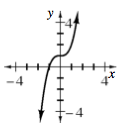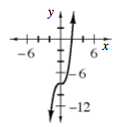### Home > A2C > Chapter 9 > Lesson 9.3.2 > Problem9-145

9-145.

Sketch the graph of each polynomial function below and find all of the zeros.

1. $y = x^{3} + 1$

Try making a table of values.$\left(−1, 0\right)$

1. $y = x^{3} − 8$

Shift the parent graph.